## 6.8The cost of simulating turbulence

The Kolmogorov microscales are the smallest scales of length, velocity and time in turbulence. The fact that the scales are so small has important consequences for CFD.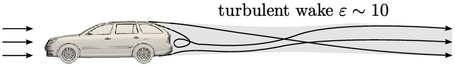In automotive external aerodynamics, boundary layers develop from the front of the vehicle which separate towards the rear. The ﬂow recirculates, forming a wake region with high levels of turbulence.

The Kolmogorov length scale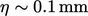, with a time scale of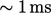, based on an estimated turbulent energy dissipation rate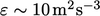and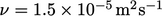for air.

To resolve the smallest eddies with CFD, a mesh with typical cell length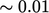mm would then be required. Since we would need to capture a wake region of volume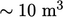, the corresponding mesh would consist of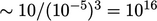cells.

In automotive external aerodynamics today (2022), some of the largest simulations run with meshes of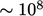cells on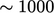processor cores. These mesh sizes are clearly several orders of magnitude smaller than those required for direct numerical simulation (DNS) of turbulence at the smallest scales.

An important consequence of using a mesh that does not resolve the smallest scales is that the main mechanism of dissipating turbulent energy is not captured. This aﬀects the rate of energy transfer within the energy cascade.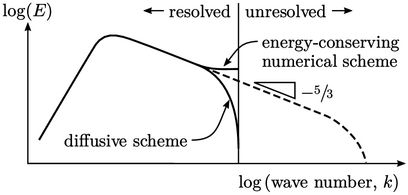If the energy dissipation is neither captured nor introduced by diﬀusive numerical schemes, excess energy inevitably “backs up”, causing over-prediction of the turbulence in the resolved eddies. Similarly, turbulence is under-predicted with diﬀusive schemes that introduce excessive numerical dissipation.

Large-eddy simulation (LES) instead uses modelling for the unresolved scales while capturing the large eddies using accurate numerical schemes. Eﬀective models dissipate the energy in the unresolved scales at a realistic rate, e.g. by the ﬁve-thirds law.

Turbulent ﬂuctuations are predicted within the resolved range of wave numbers, i.e. corresponding to lower frequencies. LES is therefore useful for problems involving excitation at those frequencies, e.g. ﬂow-induced vibration, jet breakup and noise.

The simulations are still relatively costly, however, since they are inherently transient, requiring strong convergence within each time step for accuracy. Mesh sizes are still relatively large and long simulation times are needed to generate reliable time-averaged properties.

We therefore need a faster method to calculate turbulence, suitable for ﬂows which are steady (or reasonably steady).

Notes on CFD: General Principles - 6.8 The cost of simulating turbulence